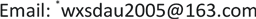1. 引言

2. 扩散映射

W ( x i , x j ) = exp ( − ‖ x i − x j ‖ 2 δ 2 ) (1)

1) 数据点x的度

d ( x ) = ∑ y ∈ X W ( x , y ) (2)

2) 转移概率

P ( x , y ) = W ( x , y ) / d ( x ) (3)

∑ y ∈ X P ( x , y ) = 1 (4)

P t ( x , y ) = ∑ l ≥ 0 λ l t φ l ( x ) ψ l ( y ) (5)

λ0为一常数，根据Mishne and Cohen  的研究，可进一步将扩散映射表示为： Y t : x → ( λ 1 t φ 1 ( x ) , λ 2 t φ 2 ( x ) , ⋯ , λ l t φ l ( x ) ) T 。

3) 扩散距离

D t ( x , y ) 2 = ∑ z ∈ X ( p t ( x , z ) − p t ( y , z ) ) 2 ϕ 0 ( z ) (6)

D t 2 ( x , y ) = ∑ j ≥ 1 λ j 2 t ( φ j ( x ) − φ j ( y ) ) 2 = ‖ ( Y ( x ) − Y ( y ) ) ‖ 2 (7)

3. 基于扩散映射的目标探测

c = 1 − exp { − 1 k ∑ t = 1 k D ( p i , p j ) / 2 σ k 1 + 3 × d position ( p i , p j ) } (8)

4. 实验及分析

5. 讨论

6. 结论及建议

1) 基于扩散映射进行数据降维，有效解决了侧扫声呐图像数据量大，Matlab计算大型矩阵效率低甚至无法进行运算的问题；

2) 利用扩散映射降维之后的扩散坐标，基于扩散距离利用目标得分方程可实现侧扫声呐图像中小目标的准确探测，且无需样本图像；

3) 目标得分方程定义方式，对目标探测结果影响很大，顾及海底复杂纹理背景差异，后续需深入研究联合图像纹理特征的目标得分方程定义公式。